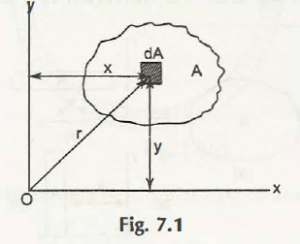Consider a thin lamina of area A and its differential area dA is shown in Fig. 7.1Suppose dA is at a distance of x from y-axis and y distance from x-axis

Its distance from point O is r

For calculating are a moment of Inertia about x-axis for dA is given by,

dIx = y2dA

Total moment of inertia can be given by,

Ix = x = 2 dA

Similarly, for y-axis, total moment of inertia is given by,

Iy = y = 2 dA

For z-axis,

Moment of inertia can be written as-

dIz = r2dA

Total moment of inertia can be given by,

Iz = z = 2 dA

Thus, we get

Ix = 2 dA

Iy = 2 dA

Iz = 2 dA

= 2 + y2) dA

= 2dA + 2 dA

= Ix + Iy

This can also be written as

i.e.,

Iz = Ix + Iy

Note:

S.I. unit of area moment of inertia is L4

Where,

L = Length (m) = m4

Links of Next Mechanical Engineering Topics:-### Customer Reviews

My Homework Help
Rated 5.0 out of 5 based on 510 customer reviews at